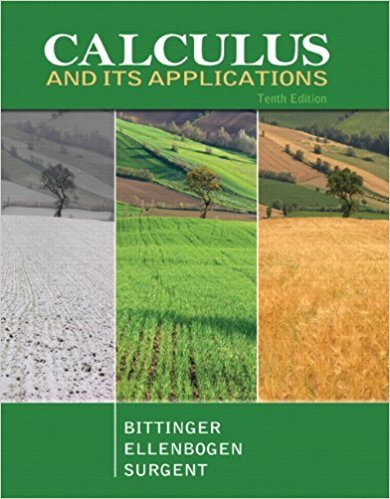×
×

# Solutions for Chapter 6.3: MaximumMinimum Problems## Full solutions for Calculus and Its Applications | 10th Edition

ISBN: 9780321694331Solutions for Chapter 6.3: MaximumMinimum Problems

Solutions for Chapter 6.3
4 5 0 315 Reviews
29
1
##### ISBN: 9780321694331

Since 32 problems in chapter 6.3: MaximumMinimum Problems have been answered, more than 26472 students have viewed full step-by-step solutions from this chapter. Calculus and Its Applications was written by and is associated to the ISBN: 9780321694331. This textbook survival guide was created for the textbook: Calculus and Its Applications, edition: 10. This expansive textbook survival guide covers the following chapters and their solutions. Chapter 6.3: MaximumMinimum Problems includes 32 full step-by-step solutions.

Key Calculus Terms and definitions covered in this textbook
• Directed line segment

See Arrow.

• Distributive property

a(b + c) = ab + ac and related properties

• Exponential decay function

Decay modeled by ƒ(x) = a ? bx, a > 0 with 0 < b < 1.

• Extraneous solution

Any solution of the resulting equation that is not a solution of the original equation.

• Geometric sequence

A sequence {an}in which an = an-1.r for every positive integer n ? 2. The nonzero number r is called the common ratio.

• Grapher or graphing utility

Graphing calculator or a computer with graphing software.

• Hyperbola

A set of points in a plane, the absolute value of the difference of whose distances from two fixed points (the foci) is a constant.

• Intercepted arc

Arc of a circle between the initial side and terminal side of a central angle.

• Lemniscate

A graph of a polar equation of the form r2 = a2 sin 2u or r 2 = a2 cos 2u.

• Logistic regression

A procedure for fitting a logistic curve to a set of data

• Negative linear correlation

See Linear correlation.

• Number line graph of a linear inequality

The graph of the solutions of a linear inequality (in x) on a number line

• One-to-one rule of logarithms

x = y if and only if logb x = logb y.

• Ordered pair

A pair of real numbers (x, y), p. 12.

• Parametrization

A set of parametric equations for a curve.

• Polar coordinates

The numbers (r, ?) that determine a point’s location in a polar coordinate system. The number r is the directed distance and ? is the directed angle

• Quotient of complex numbers

a + bi c + di = ac + bd c2 + d2 + bc - ad c2 + d2 i

• Real number

Any number that can be written as a decimal.

• Sequence

See Finite sequence, Infinite sequence.

• Slope

Ratio change in y/change in x

×